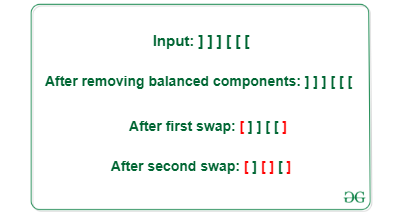Open in App
Not now

# Minimum swaps to balance the given brackets at any index

• Difficulty Level : Easy
• Last Updated : 12 Oct, 2021

Given a balanced string of even length consisting of equal number of opening brackets ‘[‘ and closing brackets ‘]’ , Calculate the minimum number of swaps to make string balanced. An unbalanced string can be made balanced by swapping any two brackets.

A string is called balanced if it can be represented in the form of “[S1]” where S1 is a balanced string

Example:

Input: s= “] ] ] [ [ [“
Output: 2
Explanation: First swap: Position 0 and 5 = [ ] ] [ [ ]
Second swap: Position 2 and 3 = [][][]Input: s= “] ] ] ] [ ] [ [ [ [“
Output: 2
Explanation: first swap for brackets at position 2 and 5, second swap for brackets at position 0 and 7

Approach: Given problem can be solved by iterating through the string and following the steps below:

• All the balanced brackets are removed as they do not require any swaps for balancing the string
• Since, the number of opening bracket ‘[‘ and closing bracket is same ‘]’, After removing balanced components , remaining string becomes like  ] ] ]…..[ [
• The optimal approach is to balance two sets of brackets in one swap
• For every two pairs of square brackets, a swap will make them balanced.
• If number of unbalanced pairs are odd, then one more swap is needed.
• If p is the number of unbalanced pairs then

minimum number of swaps = (p + 1) / 2

•

Below is the implementation of the above approach

## C++

 `// C++ implementation for the above approach` `#include ` `using` `namespace` `std;`   `// Function to balance the given bracket by swap` `int` `BalancedStringBySwapping(string s)` `{`   `    ``// To count the number of uunbalanced pairs` `    ``int` `unbalancedPair = 0;` `    ``for` `(``int` `i = 0; i < s.length(); ++i)` `    ``{`   `        ``// if there is an opening bracket and` `        ``// we encounter closing bracket then it will` `        ``// decrement the count of unbalanced bracket.` `        ``if` `(unbalancedPair > 0 && s[i] == ``']'``)` `        ``{` `            ``--unbalancedPair;` `        ``}`   `        ``// else it will increment unbalanced pair count` `        ``else` `if` `(s[i] == ``'['``)` `        ``{` `            ``++unbalancedPair;` `        ``}` `    ``}`   `    ``return` `(unbalancedPair + 1) / 2;` `}`   `// Driver code` `int` `main()` `{`   `    ``string s = ``"]]][[["``;` `    ``cout << (BalancedStringBySwapping(s));`   `    ``return` `0;` `}`   `// This code is contributed by Potta Lokesh`

## Java

 `// Java implementation for the above approach`   `import` `java.io.*;` `import` `java.util.*;`   `class` `GFG {`   `// Function to balance the given bracket by swap` `    ``static` `int` `BalancedStringBySwapping(String s)` `    ``{`   `        ``// To count the number of uunbalanced pairs` `        ``int` `unbalancedPair = ``0``;` `        ``for` `(``int` `i = ``0``; i < s.length(); ++i) {`   `            ``// if there is an opening bracket and` `            ``// we encounter closing bracket then it will` `            ``// decrement the count of unbalanced bracket.` `            ``if` `(unbalancedPair > ``0` `&& s.charAt(i) == ``']'``) {` `                ``--unbalancedPair;` `            ``}`   `            ``// else it will increment unbalanced pair count` `            ``else` `if` `(s.charAt(i) == ``'['``) {` `                ``++unbalancedPair;` `            ``}` `        ``}`   `        ``return` `(unbalancedPair + ``1``) / ``2``;` `    ``}`   `// Driver code` `    ``public` `static` `void` `main(String[] args)` `    ``{`   `        ``String s = ``"]]][[["``;` `        ``System.out.println(BalancedStringBySwapping(s));` `    ``}`   `}`

## Python3

 `# Python3 implementation for the above approach`   `# Function to balance the given bracket by swap` `def` `BalancedStringBySwapping(s) :`   `    ``# To count the number of uunbalanced pairs` `    ``unbalancedPair ``=` `0``;` `    ``for` `i ``in` `range``(``len``(s)) :`   `        ``# if there is an opening bracket and` `        ``# we encounter closing bracket then it will` `        ``# decrement the count of unbalanced bracket.` `        ``if` `(unbalancedPair > ``0` `and` `s[i] ``=``=` `']'``) :` `        `  `            ``unbalancedPair ``-``=` `1``;`   `        ``# else it will increment unbalanced pair count` `        ``elif` `(s[i] ``=``=` `'['``) :` `        `  `            ``unbalancedPair ``+``=` `1``;`   `    ``return` `(unbalancedPair ``+` `1``) ``/``/` `2``;`   `# Driver code` `if` `__name__ ``=``=` `"__main__"` `:`   `    ``s ``=` `"]]][[["``;` `    ``print``(BalancedStringBySwapping(s));`     `    ``# This code is contributed by AnkThon.`

## C#

 `// C# implementation for the above approach` `using` `System;`   `class` `GFG ` `{`   `  ``// Function to balance the given bracket by swap` `  ``static` `int` `BalancedStringBySwapping(String s)` `  ``{`   `    ``// To count the number of uunbalanced pairs` `    ``int` `unbalancedPair = 0;` `    ``for` `(``int` `i = 0; i < s.Length; ++i) {`   `      ``// if there is an opening bracket and` `      ``// we encounter closing bracket then it will` `      ``// decrement the count of unbalanced bracket.` `      ``if` `(unbalancedPair > 0 && s[i] == ``']'``) {` `        ``--unbalancedPair;` `      ``}`   `      ``// else it will increment unbalanced pair count` `      ``else` `if` `(s[i] == ``'['``) {` `        ``++unbalancedPair;` `      ``}` `    ``}`   `    ``return` `(unbalancedPair + 1) / 2;` `  ``}`   `  ``// Driver code` `  ``public` `static` `void` `Main(String[] args)` `  ``{`   `    ``String s = ``"]]][[["``;` `    ``Console.Write(BalancedStringBySwapping(s));` `  ``}`   `}`   `// This code is contributed by shivanisinghss2110`

## Javascript

 ``

Output

`2`

Time Complexity: O(N)
Auxiliary Space: O(1)

My Personal Notes arrow_drop_up
Related Articles